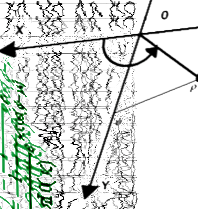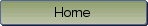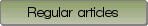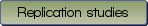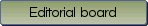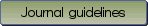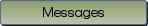Regression models for count data with excess zeros: A comparison using survey data

Bibliographic information: BibTEX format RIS format XML format APA style
Cited references information: BibTEX format APA style

Bhaskar, Adhin , Thennarasu, K. , Philip, Mariamma , Jaisoorya, T. S.
1-13
Keywords: count data; Poisson; negative binomial; zero inflation; hurdle regression
Tools: R
(no sample data)   (Appendix)

Presence of excess zeros and the distributions are major concern in modeling count data. Zero inflated and hurdle models are regression techniques which can handle zero inflated count data. This study compares various count regression models for survey data observed with excess zeros. The data for the study is obtained from a survey conducted to assess the harms attributable to drinkers among children. Poisson, negative binomial and their zero inflated and hurdle versions were compared by fitting them to two count response variables, number of physical and number of psychological harms. The models were compared using fit indices, residual analysis and predicted values. The robustness of the models were also compared using simulated data sets. Results indicated that the Poisson regression was less robust to deviations from the distributional assumptions. The negative binomial regression and hurdle regression model were found to be suitable to model the number of physical and number of psychological harms respectively. The results showed that excess zeros in count data does not imply zero inflation. The zero inflated or hurdle models are suitable for zero inflated data. The selection between the zero inflated and hurdle models should be based on the assumed cause of zeros.

Be informed of the upcoming issues with RSS feed:RSS